pyclustering.nnet.sync.sync_dynamic Class Reference

Represents output dynamic of Sync. More...Inheritance diagram for pyclustering.nnet.sync.sync_dynamic:

## Public Member Functions

def output (self)
(list) Returns output dynamic of the Sync network (phase coordinates of each oscillator in the network) during simulation.

def time (self)
(list) Returns sampling times when dynamic is measured during simulation.

def __init__ (self, phase, time, ccore=None)
Constructor of Sync dynamic. More...

def __del__ (self)
Default destructor of Sync dynamic.

def __len__ (self)
Returns number of simulation steps that are stored in dynamic. More...

def __getitem__ (self, index)
Indexing of the dynamic.

def allocate_sync_ensembles (self, tolerance=0.01, indexes=None, iteration=None)
Allocate clusters in line with ensembles of synchronous oscillators where each synchronous ensemble corresponds to only one cluster. More...

def allocate_phase_matrix (self, grid_width=None, grid_height=None, iteration=None)
Returns 2D matrix of phase values of oscillators at the specified iteration of simulation. More...

def allocate_correlation_matrix (self, iteration=None)
Allocate correlation matrix between oscillators at the specified step of simulation. More...

def calculate_order_parameter (self, start_iteration=None, stop_iteration=None)
Calculates level of global synchorization (order parameter). More...

def calculate_local_order_parameter (self, oscillatory_network, start_iteration=None, stop_iteration=None)
Calculates local order parameter. More...

## Detailed Description

Represents output dynamic of Sync.

Definition at line 113 of file sync.py.

## ◆ __init__()

 def pyclustering.nnet.sync.sync_dynamic.__init__ ( self, phase, time, ccore = None )

Constructor of Sync dynamic.

Parameters
 [in] phase (list): Dynamic of oscillators on each step of simulation. If ccore pointer is specified than it can be ignored. [in] time (list): Simulation time. [in] ccore (ctypes.pointer): Pointer to CCORE sync_dynamic instance in memory.

Definition at line 143 of file sync.py.

## ◆ __len__()

 def pyclustering.nnet.sync.sync_dynamic.__len__ ( self )

Returns number of simulation steps that are stored in dynamic.

Returns
(uint) Number of simulation steps that are stored in dynamic.

Definition at line 167 of file sync.py.

## ◆ allocate_correlation_matrix()

 def pyclustering.nnet.sync.sync_dynamic.allocate_correlation_matrix ( self, iteration = None )

Allocate correlation matrix between oscillators at the specified step of simulation.

Parameters
 [in] iteration (uint): Number of iteration of simulation for which correlation matrix should be allocated. If iternation number is not specified, the last step of simulation is used for the matrix allocation.
Returns
(list) Correlation matrix between oscillators with size [number_oscillators x number_oscillators].

Definition at line 302 of file sync.py.

## ◆ allocate_phase_matrix()

 def pyclustering.nnet.sync.sync_dynamic.allocate_phase_matrix ( self, grid_width = None, grid_height = None, iteration = None )

Returns 2D matrix of phase values of oscillators at the specified iteration of simulation.

User should ensure correct matrix sizes in line with following expression grid_width x grid_height that should be equal to amount of oscillators otherwise exception is thrown. If grid_width or grid_height are not specified than phase matrix size will by calculated automatically by square root.

Parameters
 [in] grid_width (uint): Width of the allocated matrix. [in] grid_height (uint): Height of the allocated matrix. [in] iteration (uint): Number of iteration of simulation for which correlation matrix should be allocated. If iternation number is not specified, the last step of simulation is used for the matrix allocation.
Returns
(list) Phase value matrix of oscillators with size [number_oscillators x number_oscillators].

Definition at line 258 of file sync.py.

## ◆ allocate_sync_ensembles()

 def pyclustering.nnet.sync.sync_dynamic.allocate_sync_ensembles ( self, tolerance = 0.01, indexes = None, iteration = None )

Allocate clusters in line with ensembles of synchronous oscillators where each synchronous ensemble corresponds to only one cluster.

Parameters
 [in] tolerance (double): Maximum error for allocation of synchronous ensemble oscillators. [in] indexes (list): List of real object indexes and it should be equal to amount of oscillators (in case of 'None' - indexes are in range [0; amount_oscillators]). [in] iteration (uint): Iteration of simulation that should be used for allocation.
Returns
(list) Grours (lists) of indexes of synchronous oscillators. For example [ [index_osc1, index_osc3], [index_osc2], [index_osc4, index_osc5] ].

Definition at line 194 of file sync.py.

## ◆ calculate_local_order_parameter()

 def pyclustering.nnet.sync.sync_dynamic.calculate_local_order_parameter ( self, oscillatory_network, start_iteration = None, stop_iteration = None )

Calculates local order parameter.

Local order parameter or so-called level of local or partial synchronization is calculated by following expression: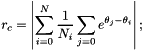where N - total amount of oscillators in the network and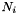- amount of neighbors of oscillator with index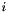.

Parameters
 [in] oscillatory_network (sync): Sync oscillatory network whose structure of connections is required for calculation. [in] start_iteration (uint): The first iteration that is used for calculation, if 'None' then the last iteration is used. [in] stop_iteration (uint): The last iteration that is used for calculation, if 'None' then 'start_iteration' + 1 is used.
Returns
(list) List of levels of local (partial) synchronization (local order parameter evolution).

Definition at line 381 of file sync.py.

## ◆ calculate_order_parameter()

 def pyclustering.nnet.sync.sync_dynamic.calculate_order_parameter ( self, start_iteration = None, stop_iteration = None )

Calculates level of global synchorization (order parameter).

This parameter is tend 1.0 when the oscillatory network close to global synchronization and it tend to 0.0 when desynchronization is observed in the network. Order parameter is calculated using following equation: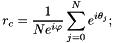where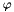is a average phase coordinate in the network,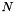is an amount of oscillators in the network.

Parameters
 [in] start_iteration (uint): The first iteration that is used for calculation, if 'None' then the last iteration is used. [in] stop_iteration (uint): The last iteration that is used for calculation, if 'None' then 'start_iteration' + 1 is used.

Example:

oscillatory_network = sync(16, type_conn = conn_type.ALL_TO_ALL);
output_dynamic = oscillatory_network.simulate_static(100, 10);
print("Order parameter at the last step: ", output_dynamic.calculate_order_parameter());
print("Order parameter at the first step:", output_dynamic.calculate_order_parameter(0));
print("Order parameter evolution between 40 and 50 steps:", output_dynamic.calculate_order_parameter(40, 50));
Returns
(list) List of levels of global synchronization (order parameter evolution).